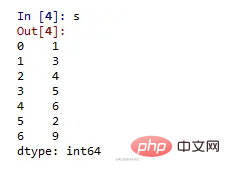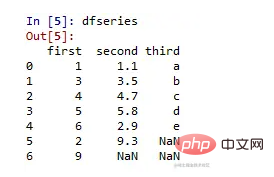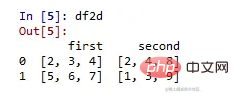# Python利用Pandas进行数据分析的方法详解

php入门到就业线上直播课：进入学习

【相关推荐：Python3视频教程

Pandas是最流行的用于数据分析的 Python 库。它提供高度优化的性能，后端源代码完全用CPython编写。

• 1.Series

• 2.数据帧

## Series

Series 是 pandas 中定义的一维（1-D）数组，可用于存储任何数据类型。

### 代码 #1

```# 创建 Series 的程序

# 导入 Panda 库
import pandas as pd

# 使用数据和索引创建 Series
a = pd.Series(Data, index = Index)```

• 一个标量值，可以是 integerValue、字符串
• 可以是键值对的Python 字典
• 一个Ndarray

### 代码 #2

```# 使用标量值创建 Series 的程序

# 数值数据
Data =[1, 3, 4, 5, 6, 2, 9]

# 使用默认索引值创建系列
s = pd.Series(Data)

# 预定义的索引值
Index =['a', 'b', 'c', 'd', 'e', 'f', 'g']

# 创建具有预定义索引值的系列
si = pd.Series(Data, Index)```### 代码#3

```# 创建词典 Series 程序
dictionary ={'a':1, 'b':2, 'c':3, 'd':4, 'e':5}

# 创建字典类型 Series
sd = pd.Series(dictionary)```### 代码 #4

```# 创建 ndarray series 的程序

# 定义二维数组
Data =[[2, 3, 4], [5, 6, 7]]

# 创建一系列二维数组
snd = pd.Series(Data)```## 数据框

DataFrames是 pandas 中定义的二维（2-D）数据结构，由行和列组成。

### 代码 #1

```# 创建 DataFrame 的程序

# 导入库
import pandas as pd

# 使用数据创建 DataFrame
a = pd.DataFrame(Data)```

• 一本或多本词典
• 一个或多个Series
• 2D-numpy Ndarray

### 代码 #2

```# 使用两个字典创建数据框的程序

# 定义字典 1
dict1 ={'a':1, 'b':2, 'c':3, 'd':4}

# 定义字典 2
dict2 ={'a':5, 'b':6, 'c':7, 'd':8, 'e':9}

# 用 dict1 和 dict2 定义数据
Data = {'first':dict1, 'second':dict2}

# 创建数据框
df = pd.DataFrame(Data)```### 代码 #3

```# 创建三个系列的Dataframe的程序
import pandas as pd

# 定义 series 1
s1 = pd.Series([1, 3, 4, 5, 6, 2, 9])

# 定义 series 2
s2 = pd.Series([1.1, 3.5, 4.7, 5.8, 2.9, 9.3])

# 定义 series 3
s3 = pd.Series(['a', 'b', 'c', 'd', 'e'])

# 定义 Data
Data ={'first':s1, 'second':s2, 'third':s3}

# 创建 DataFrame
dfseries = pd.DataFrame(Data)```### 代码 #4

```# 从二维数组创建 DataFrame 的程序

# 导入库
import pandas as pd

# 定义 2d 数组 1
d1 =[[2, 3, 4], [5, 6, 7]]

# 定义 2d 数组 2
d2 =[[2, 4, 8], [1, 3, 9]]

# 定义 Data
Data ={'first': d1, 'second': d2}

# 创建 DataFrame
df2d = pd.DataFrame(Data)```【相关推荐：Python3视频教程1/1